You have been redirected to your local version of the requested page

### Which applications benefit from using rotating electrodes?

To answer this question, we will first take a deeper look at the difference between quiescent solutions and hydrodynamic conditions. Then, after spotting the differences between laminar and turbulent flow, three main rotating electrodes and their suggested applications are highlighted.

### Quiescent solutions

The current measured at the working electrode is the result of redox reactions between electrons and reactants at the electrode–electrolyte interface. The reactants are brought to this interface by mass transport.

The mass transport is created by three processes:

1. Diffusion from concentration differences between the bulk electrolyte and the interface.
2. Migration due to the presence of an electrostatic potential. The migration is usually neglected by adding a supporting electrolyte to the solution which does not participate in the redox reaction but increases the conductivity of the electrolyte.
3. Natural convection from density changes inside the solution. This process occurs in quiescent solutions.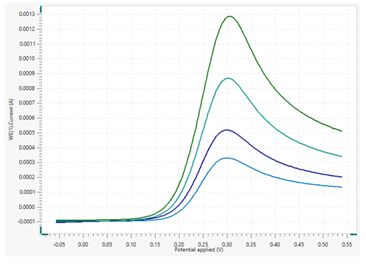Figure 1. Overlaid voltammograms of Fe(II) oxidation to Fe(III) in quiescent solution at different scan rates.

During the electrochemical oxidation of a species in solution, mass transport occurs at a rate higher than the charge transfer rate of oxidation. The charge transfer increases along with the measured current. This phenomenon occurs until the two rates reach equal values, and therefore the current reaches a maximum value. Afterwards, the mass transport is slower than the charge transfer, resulting in a decrease of current.

The voltammogram resulting from these phenomena shows a peak in the current.

For example, Figure 1 shows the resulting voltammograms of different scan rates during the oxidation of Fe+2 to Fe+3 in a quiescent ferro-ferri solution.

Here it can be seen that the higher the scan rate is, the higher the peak current.

### Hydrodynamic conditions

It is possible to force convection in the cell by rotating the working electrode. The rotation induces a swirling motion in the electrolyte. The forced convection increases the mass transport of reactants at the interface and, in parallel, removes the products from the interface.

The flow of the electrolyte resulting from the rotation can be classified as laminar or turbulent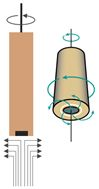Figure 2. Illustration of the laminar flow at the surface of a rotating electrode (left: cross section, right: front diagonal view).

#### Laminar flow

The laminar flow is characterized by the fluid moving in layers. Each layer moves between the adjacent layers with little or no mixing. In Figure 2, a schematic of laminar flow is shown in relation to a rotating electrode.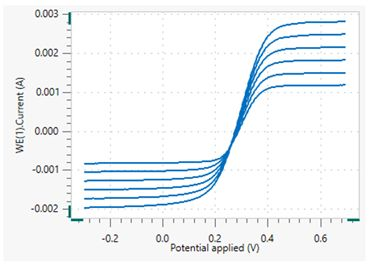Figure 3. Voltammogram of Fe(II) oxidation to Fe(III) under hydrodynamic conditions at different rotation rates.

During an electrochemical reaction under hydrodynamic conditions with laminar flow, the current increases until the mass transport occurs at a rate faster than the reaction rate. The current eventually reaches a limiting value where the rate of the redox reaction and the rate of mass transport are equal, resulting in a plateau in the voltammogram. This limiting value remains constant until the reaction is complete. The limiting current is proportional to the rotation rate of the electrode, as shown in Figure 3, where the oxidation of Fe+2 to Fe+3 under hydrodynamic conditions is investigated.

In this case, the higher the rotation rate is, the higher the limiting current.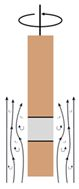Figure 4. Illustration of the turbulent flow forming at the sides of a rotating electrode.

#### Turbulent flow

Turbulent flow is the result of chaotic changes in flow velocity and pressure. It is present at the sides of a rotating electrode (Figure 4).

The turbulent flow created by measurements using rotating electrodes recreates similar conditions to those found in a pipeline, for example.

The following section focuses on different types of rotating electrodes and their suggested applications: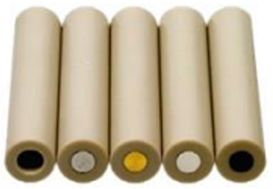Figure 5. Selection of rotating disk electrodes.

### Rotating Disk Electrode

The rotating disk electrode (RDE) is a cylinder with a disk used as the active surface. This disk is composed of a metal, glassy carbon, or an alloy (Figure 5).

Glassy carbon is used in electrocatalysis since it is an inert electrode for hydrogen reduction and supports catalysts adsorbed or deposited on its surface.

RDEs are employed to generate laminar flow, and are often used in fundamental electrochemistry experiments to investigate the properties of electrolytes. They are also utilized in electrocatalysis studies to measure the performance of catalysts and in sensors to investigate the detection mechanism.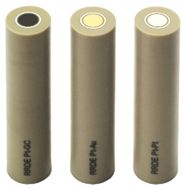Figure 6. Example of different rotating ring disk electrodes.

### Rotating Ring Disk Electrode

The rotating ring disk electrode (RRDE) is a cylinder with two active surface areas which both act as working electrodes (Figure 6). One working electrode is a disk made of platinum, gold, or glassy carbon. The second working electrode is a ring of platinum.

Like the RDEs discussed in the previous section, RRDEs are also employed to generate laminar flow. Researchers use RRDEs mainly in electrocatalysis experiments to measure the performances of different catalysts. RRDEs are also used to study reaction mechanisms. For example, the production of hydrogen peroxide during the oxygen reduction reaction is studied by detecting reaction intermediates. The RRDE also plays an important role in the study of electroplating.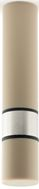Figure 7. Side view of a rotating cylinder electrode.

### Rotating Cylinder Electrode

The rotating cylinder electrode (RCE) is a cylinder with a metallic insert that serves as its active surface (Figure 7).

RCEs are used mainly in corrosion studies to exploit the turbulent flow generated along the RCE, since there is a similarity between the turbulent flow along the RCE and the turbulent flow inside a pipeline of specific thickness and diameter. For example, one common use of the RCE is in the petrochemical industry to investigate the effect of different corrosion inhibitors on the pipelines, either by using linear polarization (LP) or electrochemical impedance spectroscopy (EIS) techniques.

### Conclusion

Electrochemical studies requiring hydrodynamic conditions can be performed with rotating working electrodes to create forced convection in the measurement cell. Both laminar flow and turbulent flow conditions are able to be created in laboratory settings in order for researchers to perform different studies. The Rotating Disk Electrode (RDE) and Rotating Ring Disk Electrode (RRDE) are suitable for creating laminar flow, while the Rotating Cylinder Electrode (RCE) is the choice for making turbulent flow conditions.

RDEs are commonly used to study electrolyte properties, catalyst performance, and to investigate the detection mechanism in sensors. RRDEs are also used to study catalyst performance as well as electroplating and reaction mechanisms. RCEs are mostly used in pipeline corrosion studies and for investigating the behavior of protective coatings.